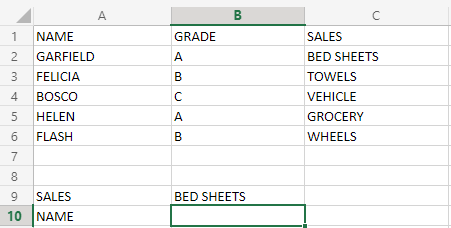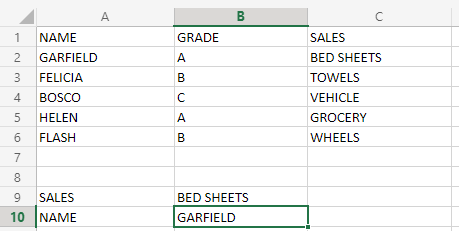GeeksforGeeks App
Open AppBrowser
Continue

# Using CHOOSE Function along with VLOOKUP in Excel

In Excel, managing space is a very important point to consider. Now, let’s suppose you are in a situation where you want to compare multiple columns in the Excel dataset, and you want to derive results, This is not possible using the VLOOKUP function alone. So, to make a function capable of doing something like this, we will introduce a special function known as CHOOSE function. But, if you don’t want to use CHOOSE function, then you have to use a helper column, making the job a little hectic.

So, in this article, we will see how to use VLOOKUP along with CHOOSE function.

First, we will see the syntax of CHOOSE FUNCTION in brief.

### CHOOSE FUNCTION:

Syntax:

`=CHOOSE(ARRAY DIMENSIONS(INDEX VALUE(S)),VALUE(1),VALUE(2),VALUE(3),.....)`

Here,

1.  Here we will define array dimensions, ie how many columns should the array have.
2. The next parameter(s) will define what value will go in the subsequent array columns, ie VALUE1 will get filled in the first column, and so on.

Example:Here we will use this dataset. Let’s suppose we have people in a sales firm, and they are evaluated for bonuses. Now, we want to know who did sales for BED SHEETS, by using VLOOKUP and CHOOSE function. So, first of all, let’s see what the formula is, and then we will understand it part by part.

`=VLOOKUP(B9,CHOOSE({1,2},C2:C6,A2:A6),2,TRUE)`

Here the output will be as shown below:Now, we will see part by part how this formula worked:

•  B9: This is the targeted value to be compared by VLOOKUP to get the result, ie BED SHEETS.
• CHOOSE({1,2},C2:C6,A2:A6): This will create an array,having first column values from C2 TO C6,and second column values from A2 TO A6.
• 2: The value will be fetched from this column number.
• TRUE: This parameter is for enabling approximate search, ie any keyword found nearly matching with the required word will be given as a result.
• VLOOKUP(B9, CHOOSE({1,2}, C2:C6, A2:A6),1, TRUE): VLOOKUP will search for the desired keyword from the second column of the array, created by CHOOSE function.

Note: The formula above will use an array, so to execute it use Ctrl+Shift+Enter key combination.

The disadvantage of the above function is that VLOOKUP is not capable of doing a case-sensitive search, so to enhance VLOOKUP you have to make it case sensitive. For reference visit this article.

My Personal Notes arrow_drop_up